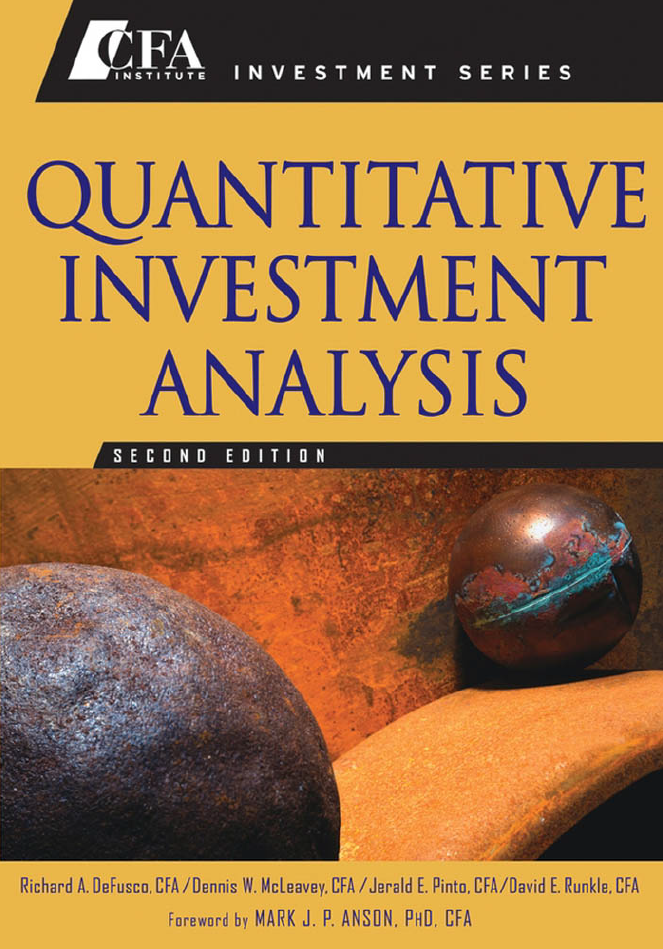23 MAR 2021

# Time Series

A time series is a set of observations about the performance of a variable over different time periods: quarterly sales for a particular company over the past five years, for example, or daily returns on a traded currency pair. Here we can explore two of the main uses of time series models: to explain the past and predict the future of a time series, and from there to orient ourselves to understand the behaviors of a given collection of data.
Some fundamental questions arise in time series analysis:

How do we predict the future value of a time series based on its past values?
How we model seasonality?
How do we choose between the time series models?
How do we model changes in the variance of the time series over time?

In many cases, the goal of studying time series is to apply a linear regression to a given time series. Unfortunately, working with time series we often find that linear regression model assumptions are not satisfied. To apply the time series analysis, we must therefore ensure that the assumptions of the linear regression model are satisfied.
In many cases we can transform the time series, or specify the regression model differently, to satisfy the assumptions of the regression model linear.

Formulas and knowledge are taken from:
DeFusco, Richard A. Quantitative Investment Analysis. Wiley, 2007.